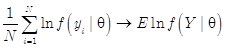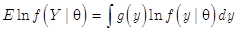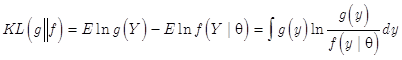# KL散度与极大似然KL-divergence，俗称KL距离，常用来衡量两个概率分布的距离。

H(X)=∑x∈XP(x)log[1/P(x)]=-x∈XP(x)log（p(x)）

DKL(Q||P)=∑x∈XQ(x)[log(1/P(x))] - ∑x∈XQ(x)[log[1/Q(x)]]=∑x∈XQ(x)log[Q(x)/P(x)]

DKL(Q||P) = -∑x∈XQ(x)log[P(x)/Q(x)] = E[-logP(x)/Q(x)] ≥ -logE[P(x)/Q(x)] = -log∑x∈XQ(x)P(x)/Q(x) = 0

===========================

KL散度是不对称的，当然，如果希望把它变对称，

Ds(p1, p2) = [D(p1, p2) + D(p2, p1)] / 2

05-30518411-086595
06-072万+
04-191210
04-16587
08-27454
09-18882
02-161074
08-145075
06-273753
12-0377
07-183603
11-081075
02-20472
08-072413
01-042868
03-267万+
09-273976
03-06666
©️2020 CSDN 皮肤主题: 编程工作室 设计师:CSDN官方博客